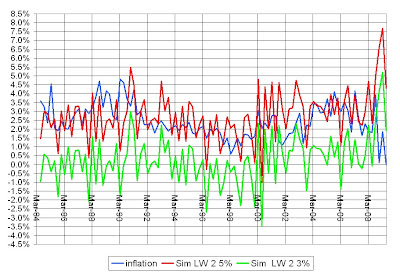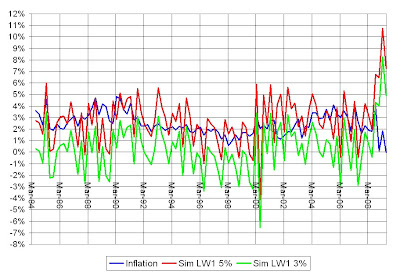## Monday, January 25, 2010

### Nominal Expenditure Targeting and Estimates of Potential Output

Suppose the Fed had been able to keep nominal expenditure growing on a 5 percent growth path? If the productive capacity of the economy had remained on the 3 percent trend growth path of real output, then the inflation rate would have stayed 2 percent.

Suppose that instead the Fed had been able to keep nominal expenditure growing on a 3 percent growth path? If the productive capacity of the economy had remained on the 3 percent trend growth path of real output, then the price level would have remained stable. There would have been no inflation.

Of course, the productive capacity of the economy, potential output, is unlikely to remain on a constant 3 percent growth path. That new technology would improve total factor productivity the same proportion each and every year is implausible. And the world is full of events that surely impact the productive capacity of the economy in both favorable and unfavorable ways--good weather, floods, hurricanes, strikes, the list is long.

The CBO provides an estimate of potential output. Using that estimate, how would the inflation rate have fared if the Fed had kept nominal expenditure on its trend growth path or else a 3% growth path? The price level is simulated by dividing the level of nominal expenditure by the estimate of potential output. The inflation rate is then calculated using log differences.If the Fed had maintained nominal expenditure on its approximately 5 percent growth path, then the highest inflation rate is approximately 3.5 percent during the first quarter of 2009. With the alternative 3 percent growth path, the highest inflation rate would be approximately 1.1 percent in the first quarter of 2009. The lowest deflation rate would have been -.7 percent, in the first quarter of 2001.Focusing on the Great Recession, it is interesting that the inflation rate consistent with nominal expenditure remaining on its trend growth rate and the CBO measure of potential output is close to 3 percent, rising to 3.5 percent. Calls for the Fed to raise its target for inflation from 2 percent to 3 percent are roughly consistent with nominal expenditure targeting and the CBO estimate of potential output.

Laubach and Williams estimate much larger fluctuations in potential output than the CBO. Their 2 way measure implies much greater fluctuations in inflation if nominal expenditure remained on a stable growth path.If nominal expenditure had continued to grow at its trend, and potential output remained at the estimated level, the highest inflation rate would have been 7.7 percent in the first quarter of 2009. If the growth path for nominal expenditures had instead been 3 percent, then the highest
inflation rate would have been 5.2 percent, also in the first quarter of 2009. The lowest deflation rate would have been -3.5 percent in the second quarter of 2000.This is quarterly inflation at annual rates. The annual rates of inflation are slightly less alarming.Still, with nominal expenditure growing at trend, the annual inflation rate for 2009 was nearly 6 percent. And even with the 3 percent growth rate of nominal expenditures, the inflation rate was 3.5 percent. These figures are both for the first half of the year, because Laubach and Williams only provide estimates for potential output for the first two quarters. The lowest annual deflation rate was approximately -1 percent in 1998.

The one way estimate from Laubach and Williams has even less attractive consequences for targeting a growth path of nominal expenditure.If nominal expenditure had been kept on its 5% trend growth path, then Laubach and William's one way estimate results in a double-digit inflation rate--10.7 percent in the first quarter of 2009. The lowest deflation rate would have been -4.1 percent in the second quarter of 2000. If nominal expenditure had been restricted to a 3 percent growth path, then inflation would have still been 8.25 percent in the first quarter of 2009. The lowest deflation rate would have been -6.5 percent.These are annualized quarterly rates of change. Looking at the annual inflation rate is only slightly less alarming.The annual inflation rate for 2009 is nearly 9 percent if nominal expenditure grew at trend and 6.6 percent with the noninflationary trend of 3 percent. The deflation rate would have been -1.5 in 1999.

The CBO estimate of potential output suggests that a stable growth path of nominal expenditure would be associated with only modest fluctuations in the price level. On the other hand, the Laubach and Williams' measures show substantial fluctuations in the price level--very sharp quarterly inflation and deflation rates.

To the degree such large fluctuations in prices are foreseen, the result should be inventory investment or disinvestment that would tend to dampen these sharp changes. Are Laubach and Williams' estimates accurate? Would they hold up under a regime of nominal expenditure targeting? Perhaps giving it a try is the best approach.

Trying to manipulate nominal expenditure in order to stabilize the price level or inflation rate requires an estimate of potential output. For example, the Taylor rule includes an estimate of potential output to determine the target for the federal funds rate. Stabilizing the growth path of nominal expenditures and so, nominal incomes, while letting the price level adjust with shifts in the growth of potential output is the more reasonable course when estimates of potential output are uncertain.

1.The rate of inflation is important,since it is subtracted from various economic rates in order to eliminate the impact of inflation.The real increase in wages is also counted by taking into account the prevailing inflation rate.

2.If there is a decrease in the productive capacity of the economy, usually, I would think this would require a decrease in real wages. If nominal wages continue to growth with other nominal incomes at the targeted growth rate of nominal expenditures, then the higher inflation reduces real wages, exactly as needs to be done.

I suppose if the reason for the decrease in the productive capacity was a decrease in labor supply, real wages would need to rise. How likely is that?

3.These are the type of things that we got to be good at; if we really wish to be successful then we need to learn about these stuff very well. I am lucky enough to have broker like OctaFX since they always keeps me updated with everything and allows me to succeed, it is all thanks to their daily market news and analysis service, it is easy to follow but got seriously high effective that helps me trade well with ease.